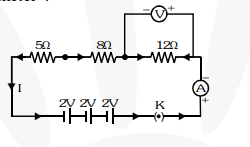# Redraw the circuit of Q. 1,Question.
Redraw the circuit of Q. 1, putting in an ammeter to measure the current through the resistors and a voltmeter to measure the voltage across the 12 ohm resistor. What would be the readings in the ammeter and the voltmeter ?solution:

Since all the three resistances are in series, total resistance in the circuit,

$R=5+8+12=25 \Omega$

Current in the circuit,

$\mathrm{I}=\frac{\mathrm{V}}{\mathrm{D}}=\frac{2+2+2}{25}=\frac{6}{25}=0.24 \mathrm{~A}$, thus, ammeter will read $0.24 \mathrm{~A} .$

Potential difference across $12 \mathrm{ohm}$ resistor, $\mathrm{V}=\mathrm{I} \times \mathrm{R}=0.24 \times 12=2.88 \mathrm{~V}$